# NCERT Solutions Class 10 Maths Chapter 5 Arithmetic Progressions

Here you can get free NCERT Solutions for Class 10 Maths, of Chapter 5 Arithmetic Progressions. All NCERT Book Solutions are given here exercise wise for Arithmetic Progressions. NCERT Solutions are helpful in the preparation of several school level, graduate and undergraduate level competitive exams. Practicing questions from NCERT Maths Book, its Solutions for Class 10 Chapter 5 Arithmetic Progressions is proven to enhance your subject skills.

 Class: 10th Class Chapter: Chapter 5 Name: Arithmetic Progressions Subject: Maths

## NCERT Solutions Class 10 Maths Chapter 5 – Arithmetic Progressions

NCERT Class 10 Solutions Chapter 5 – Arithmetic Progressions are given below.

NCERT Solutions for Class 10 Maths Chapter 5 Arithmetic Progression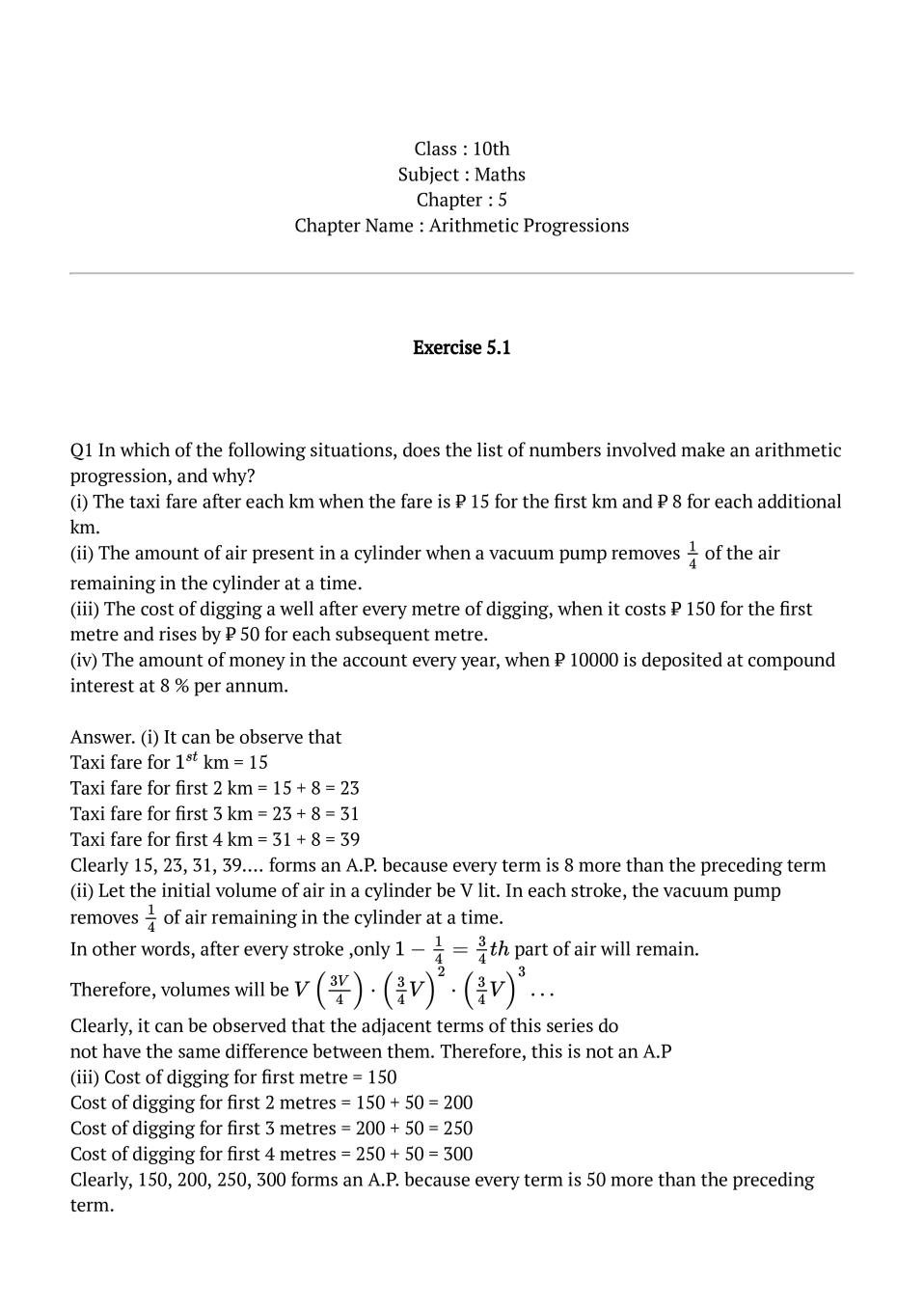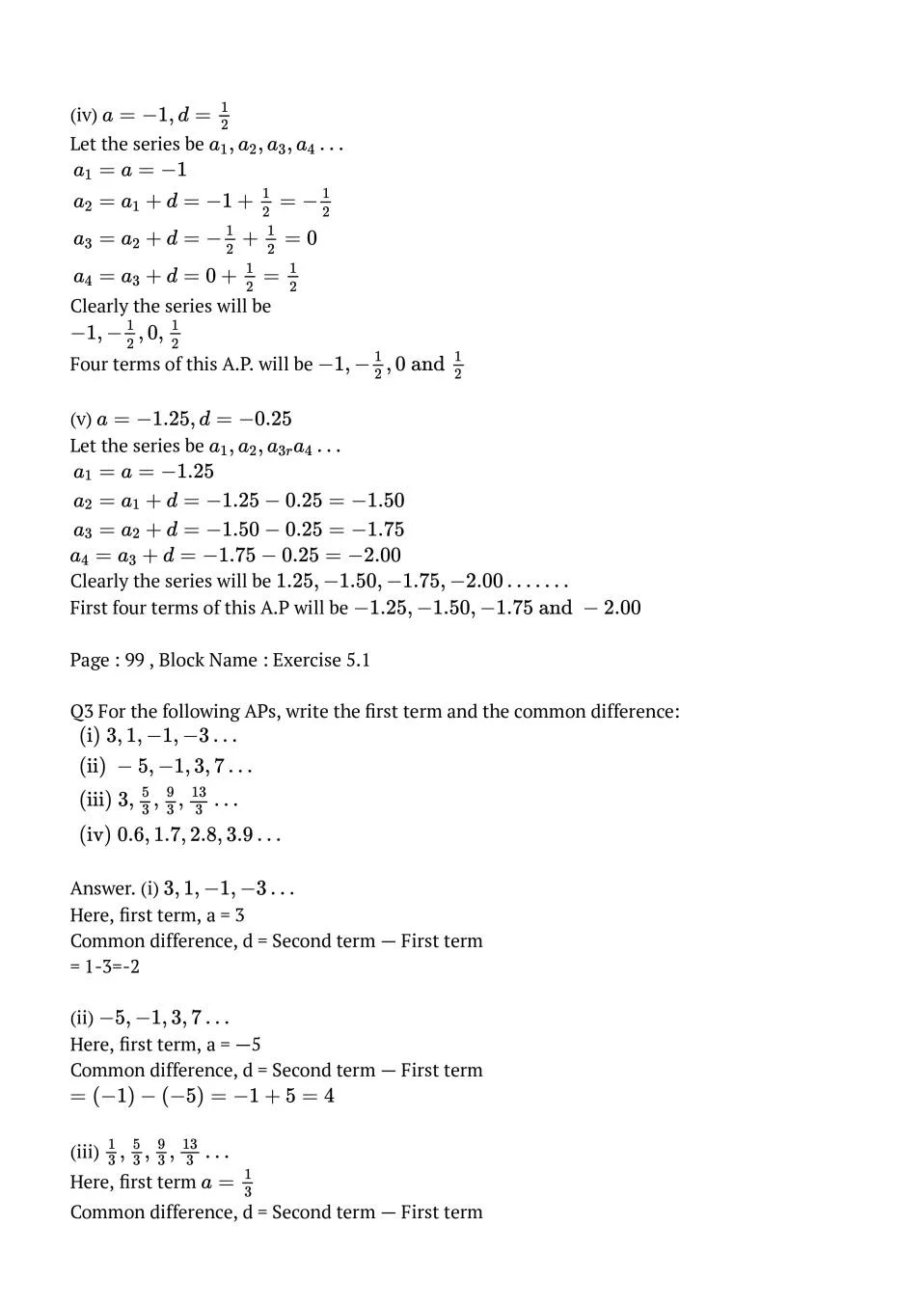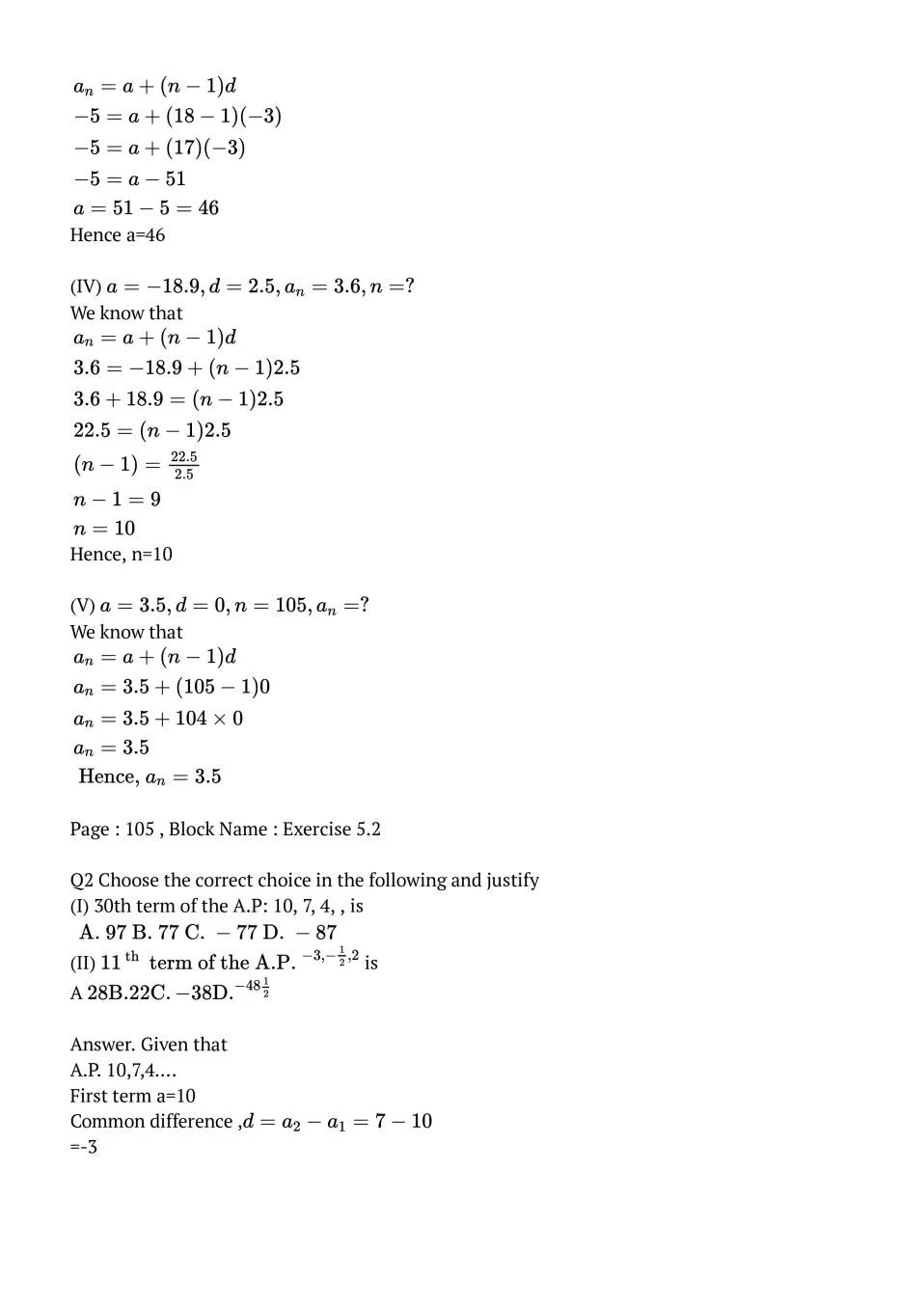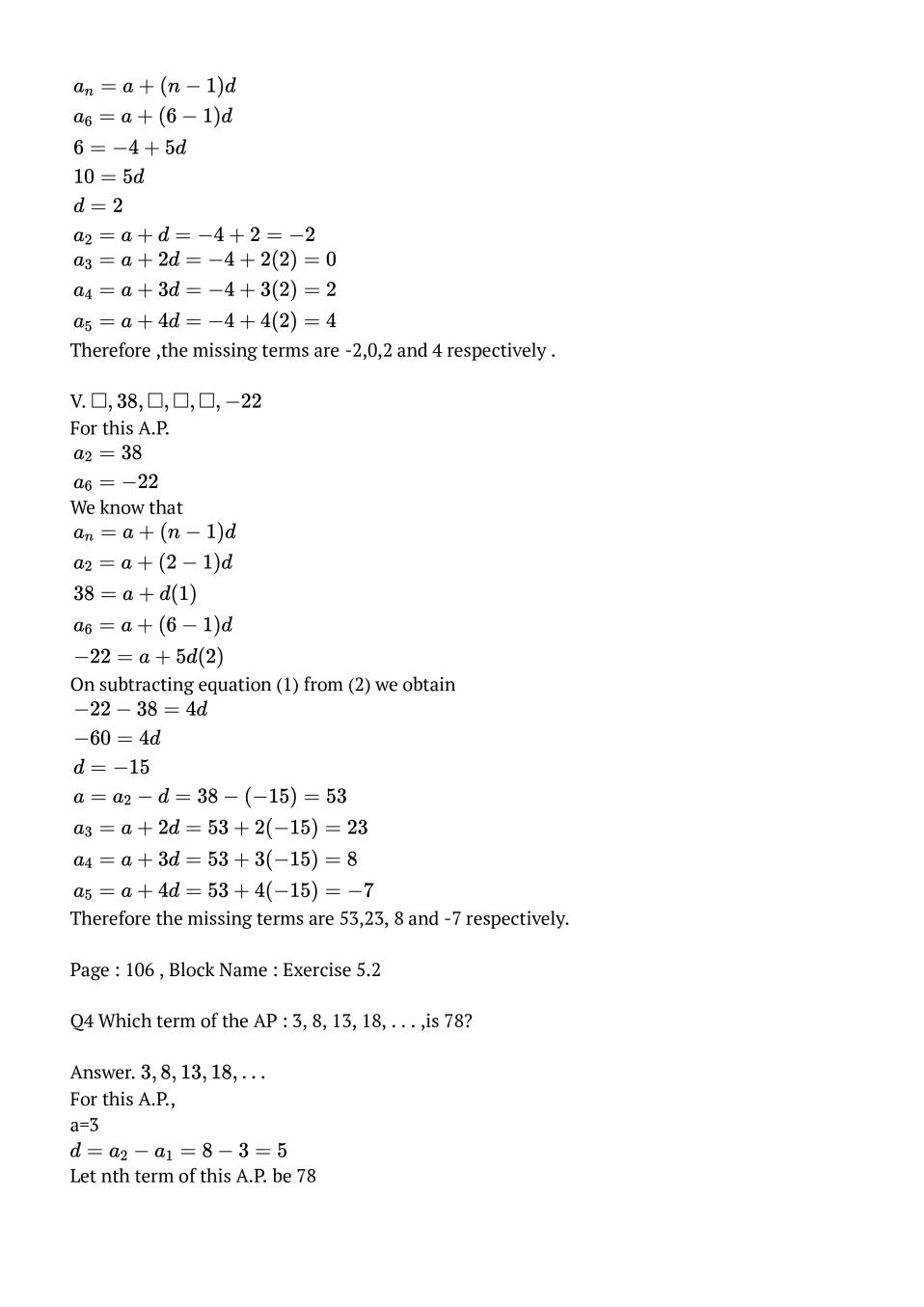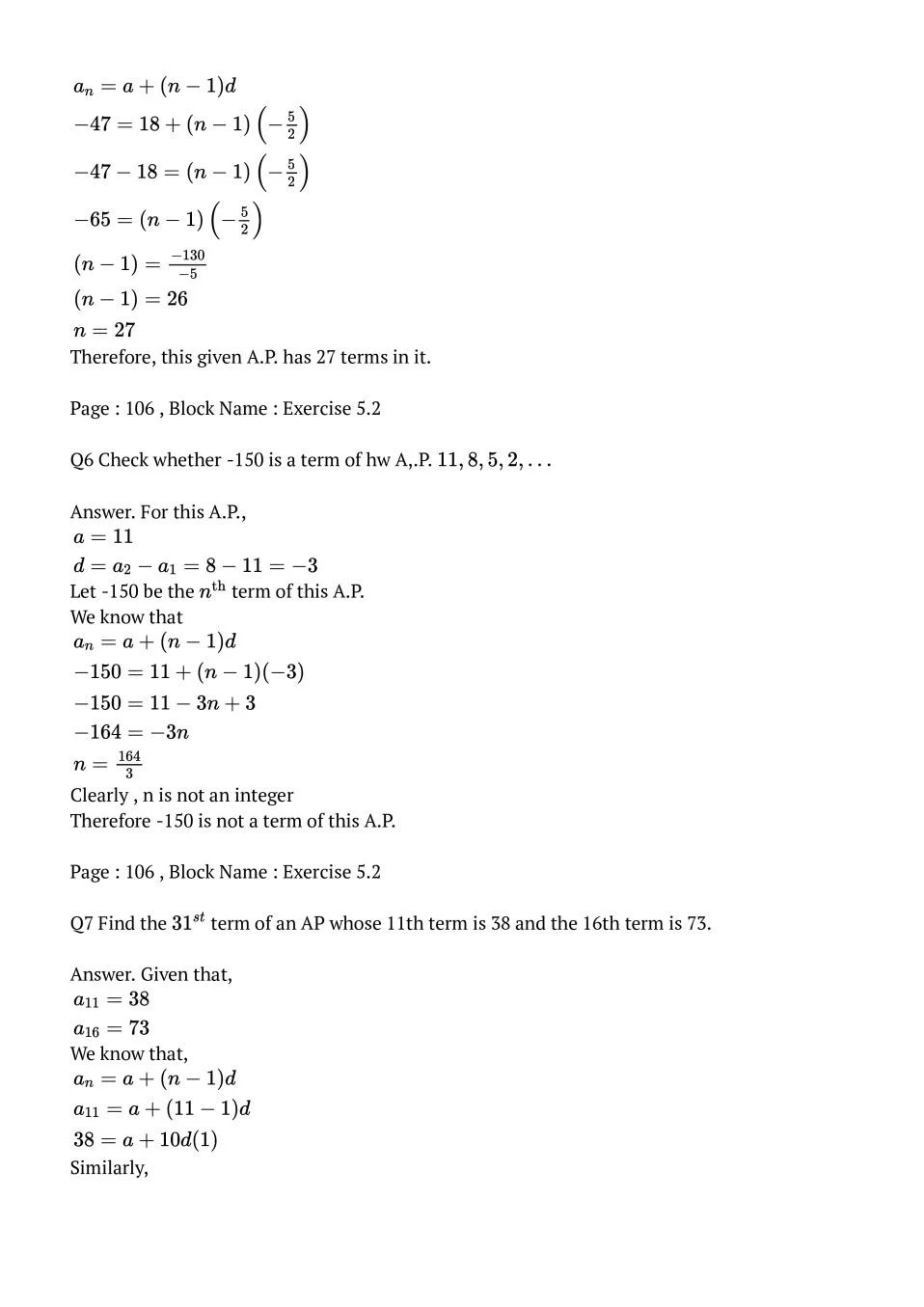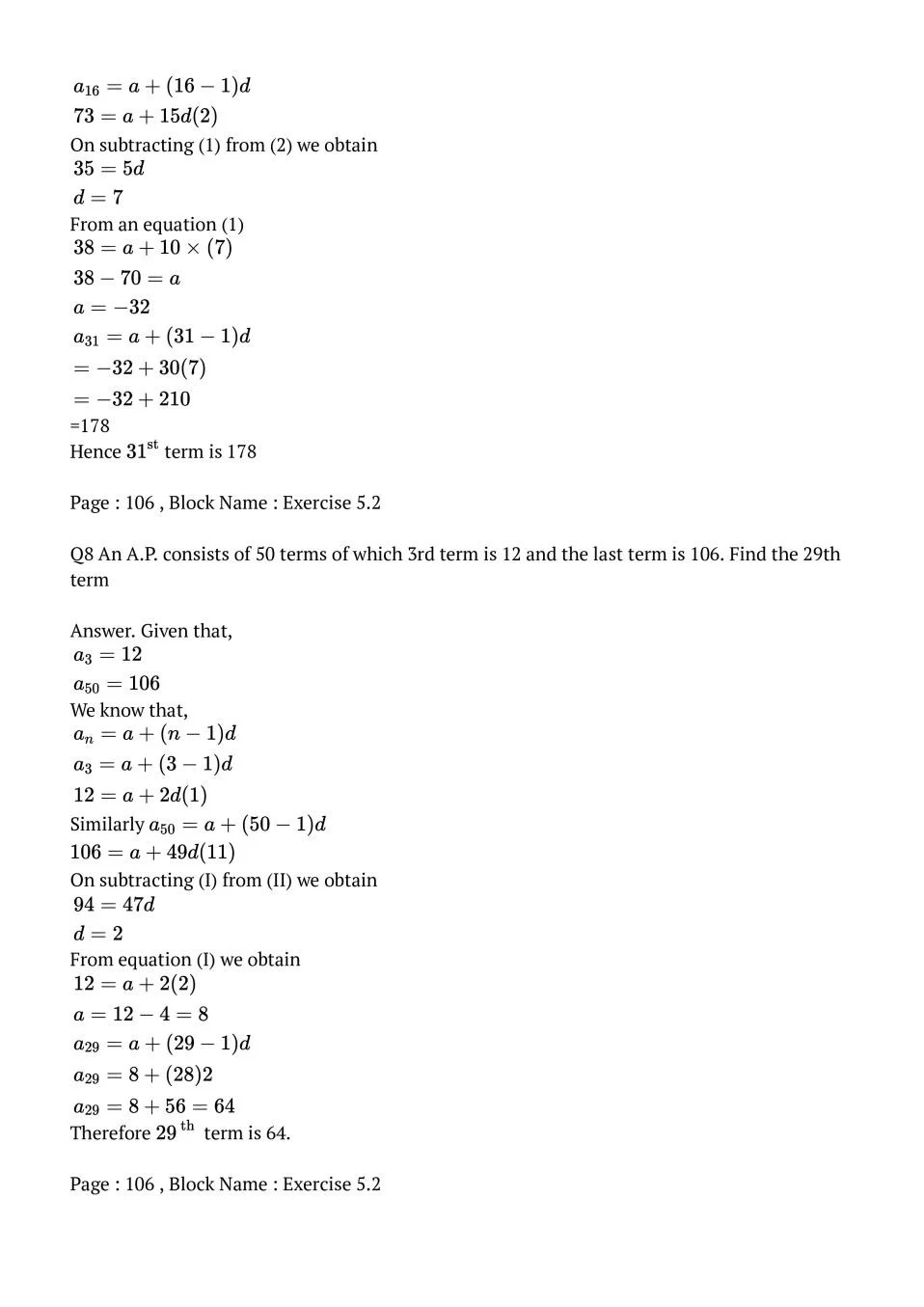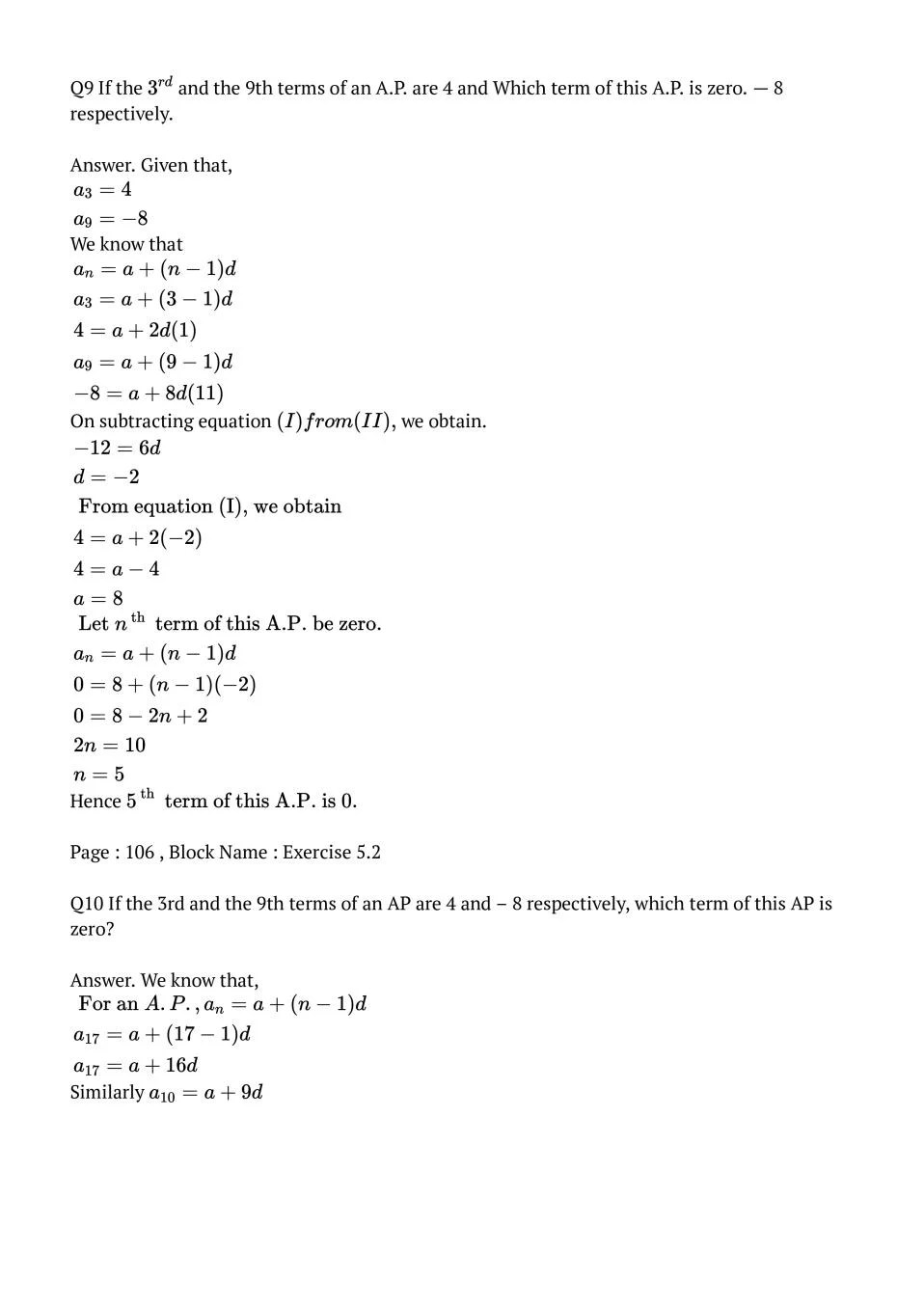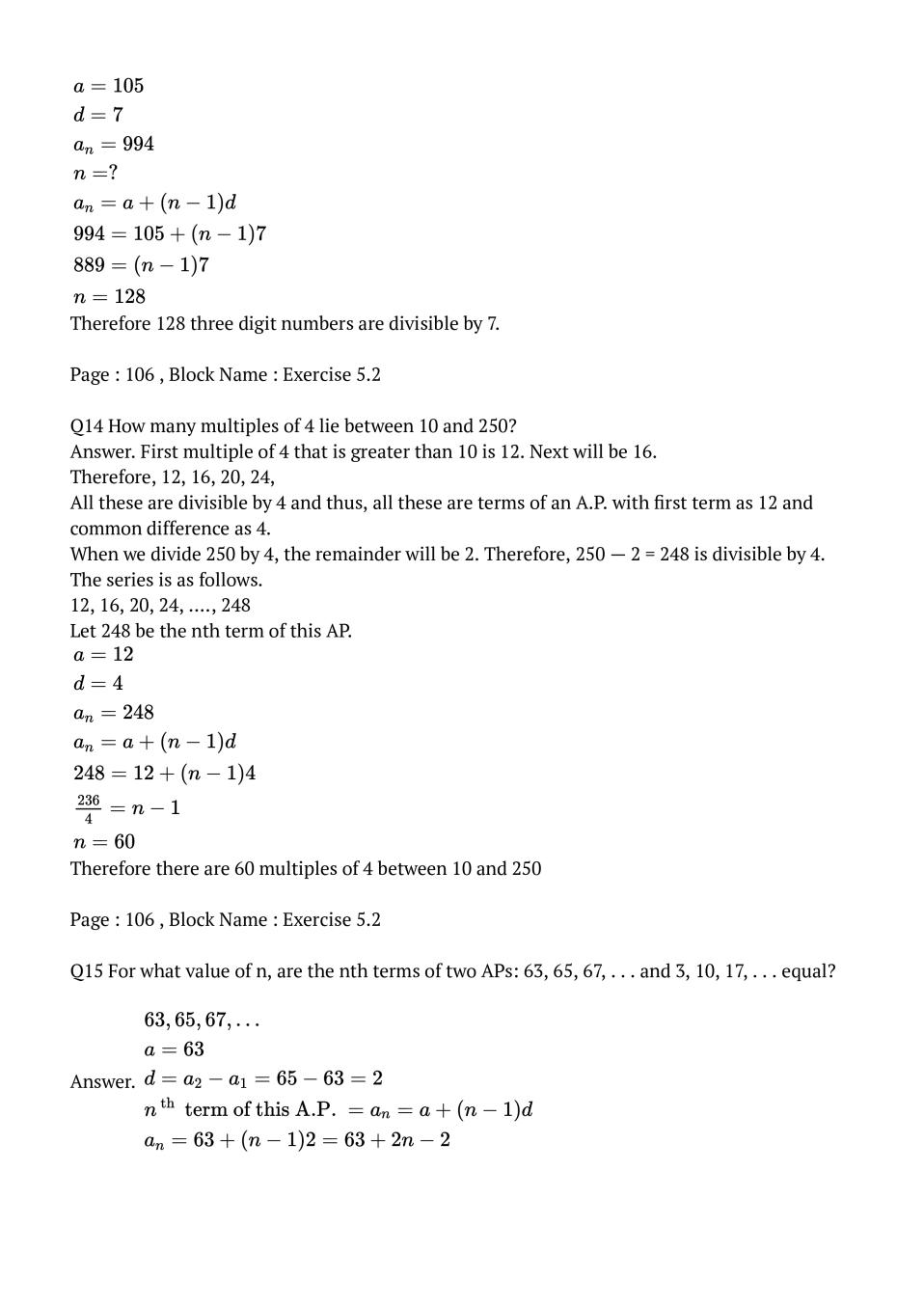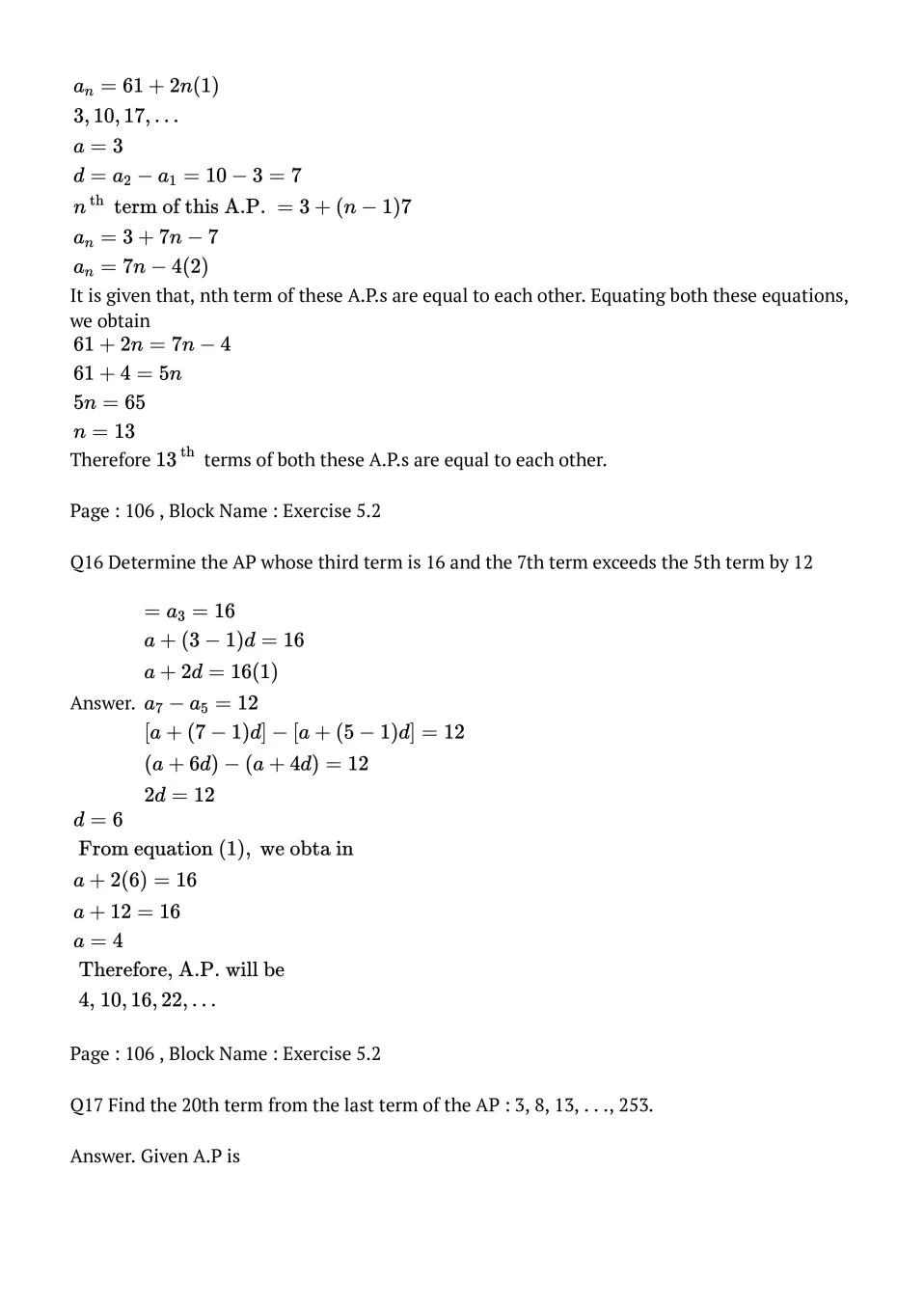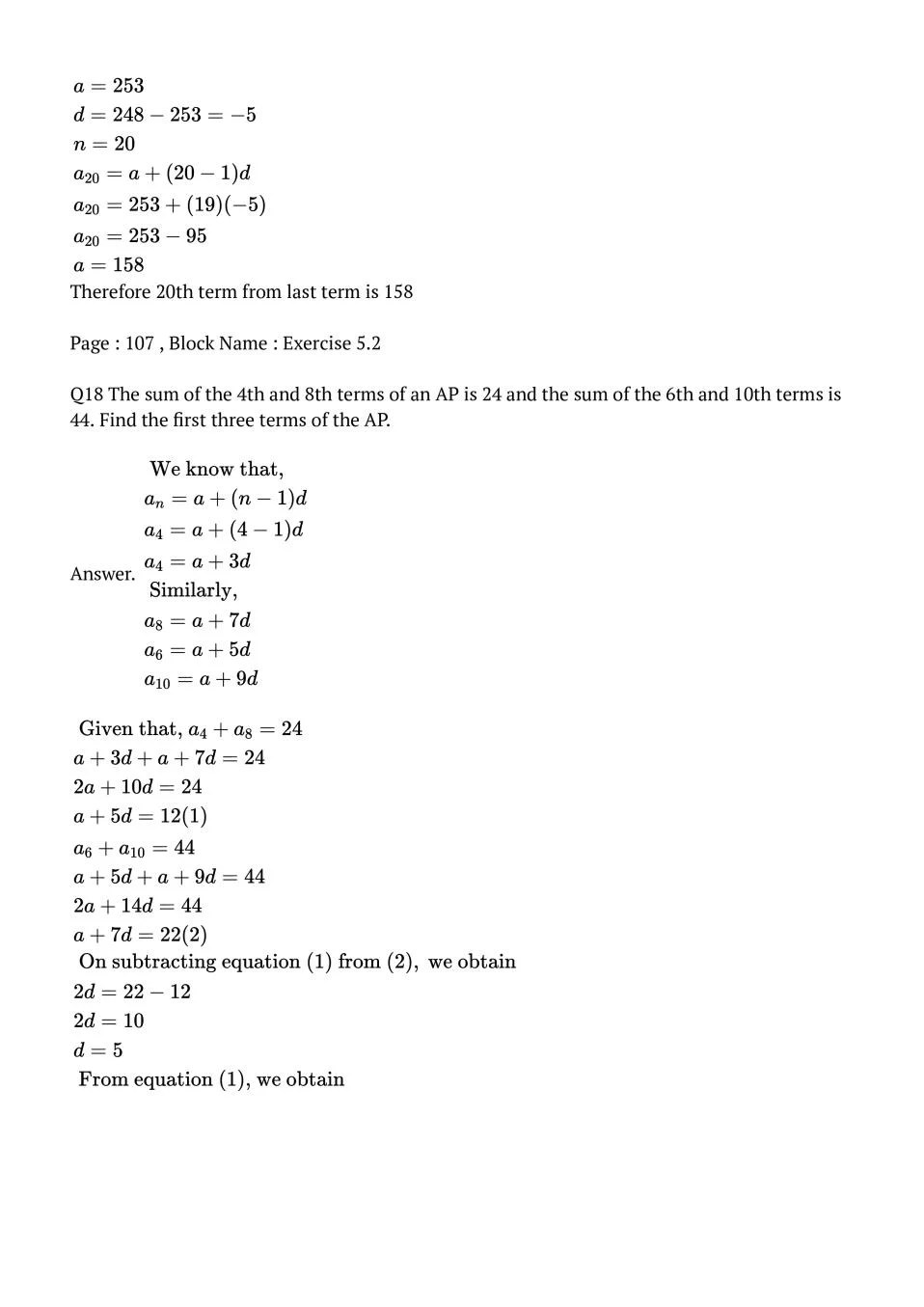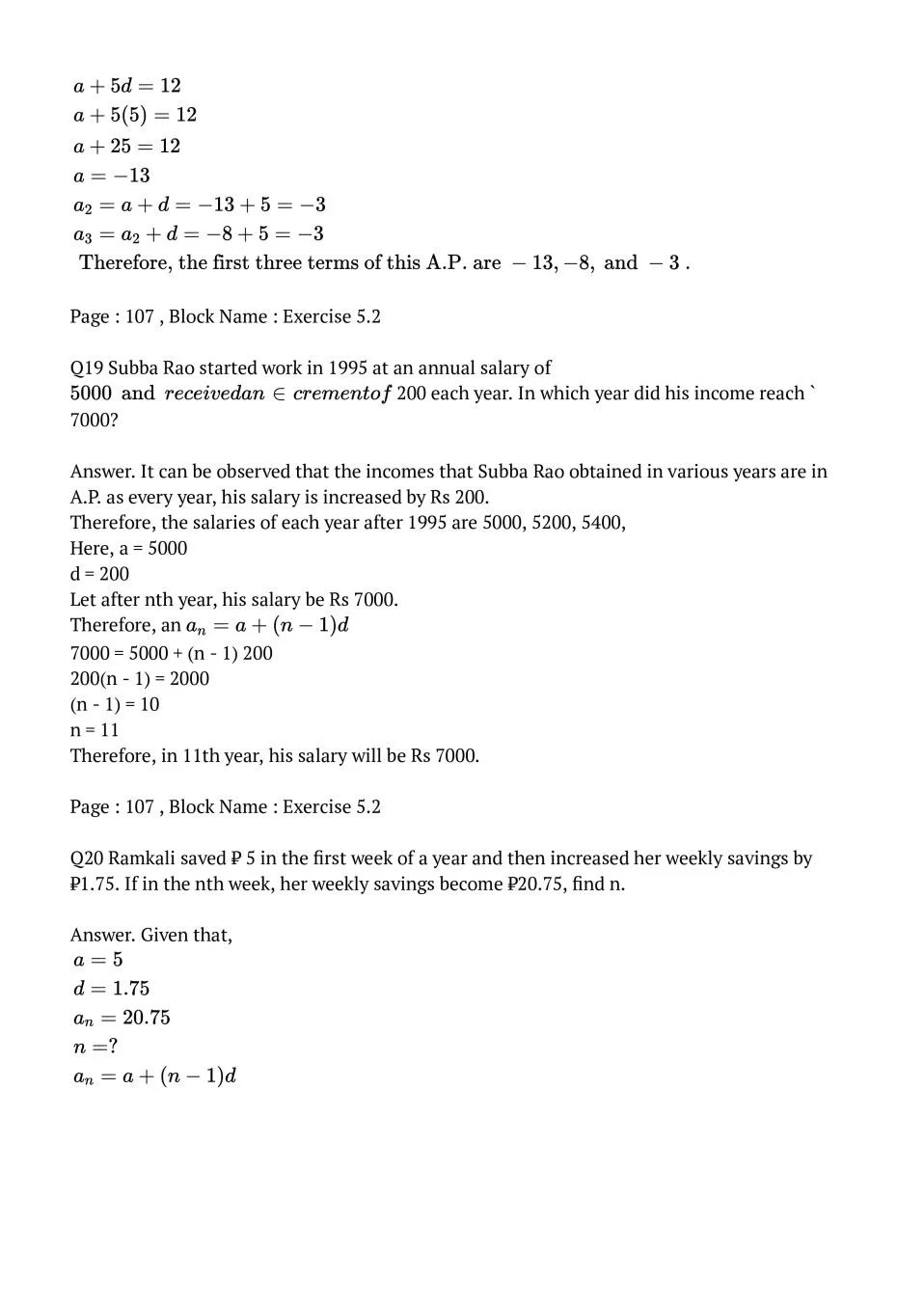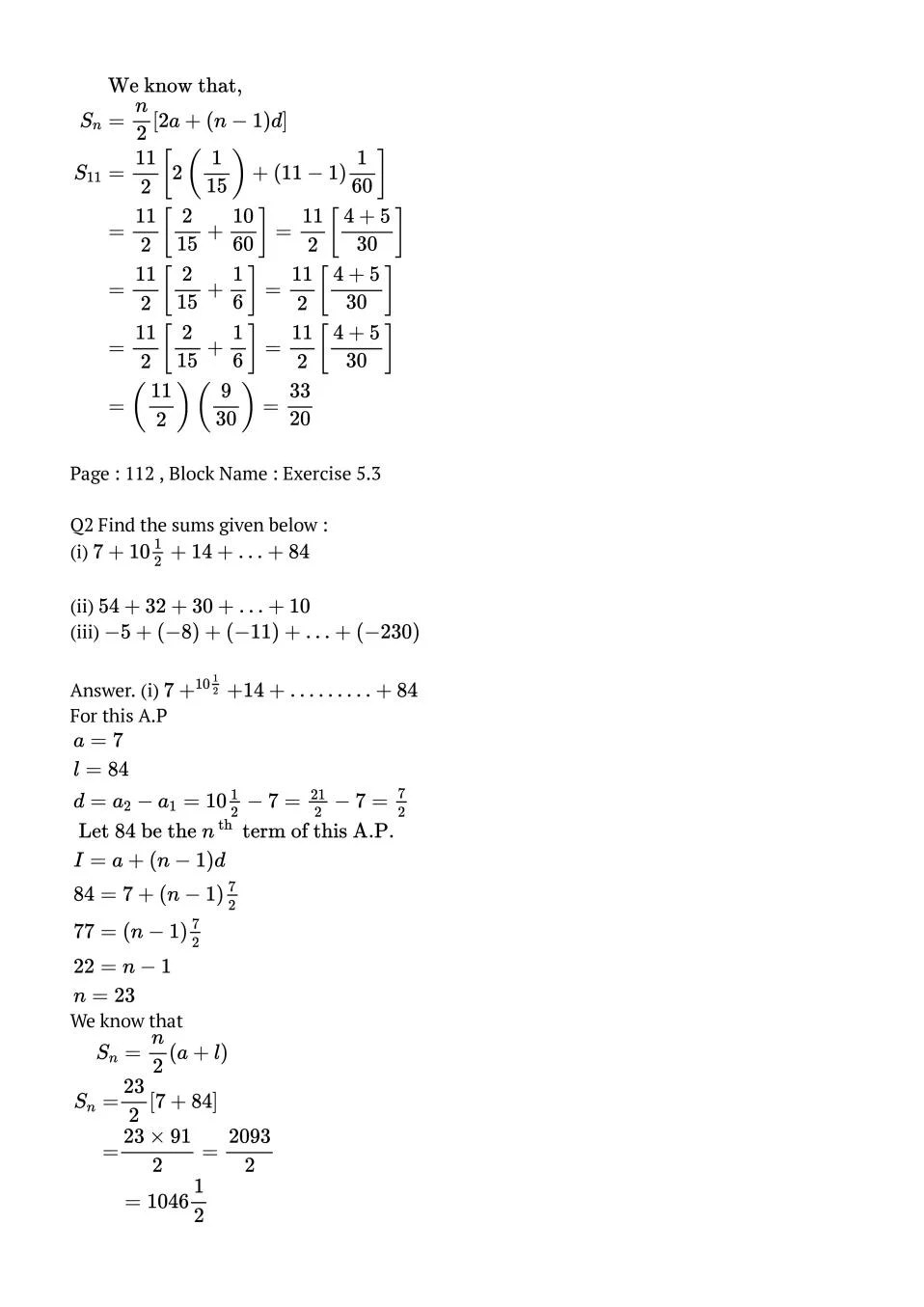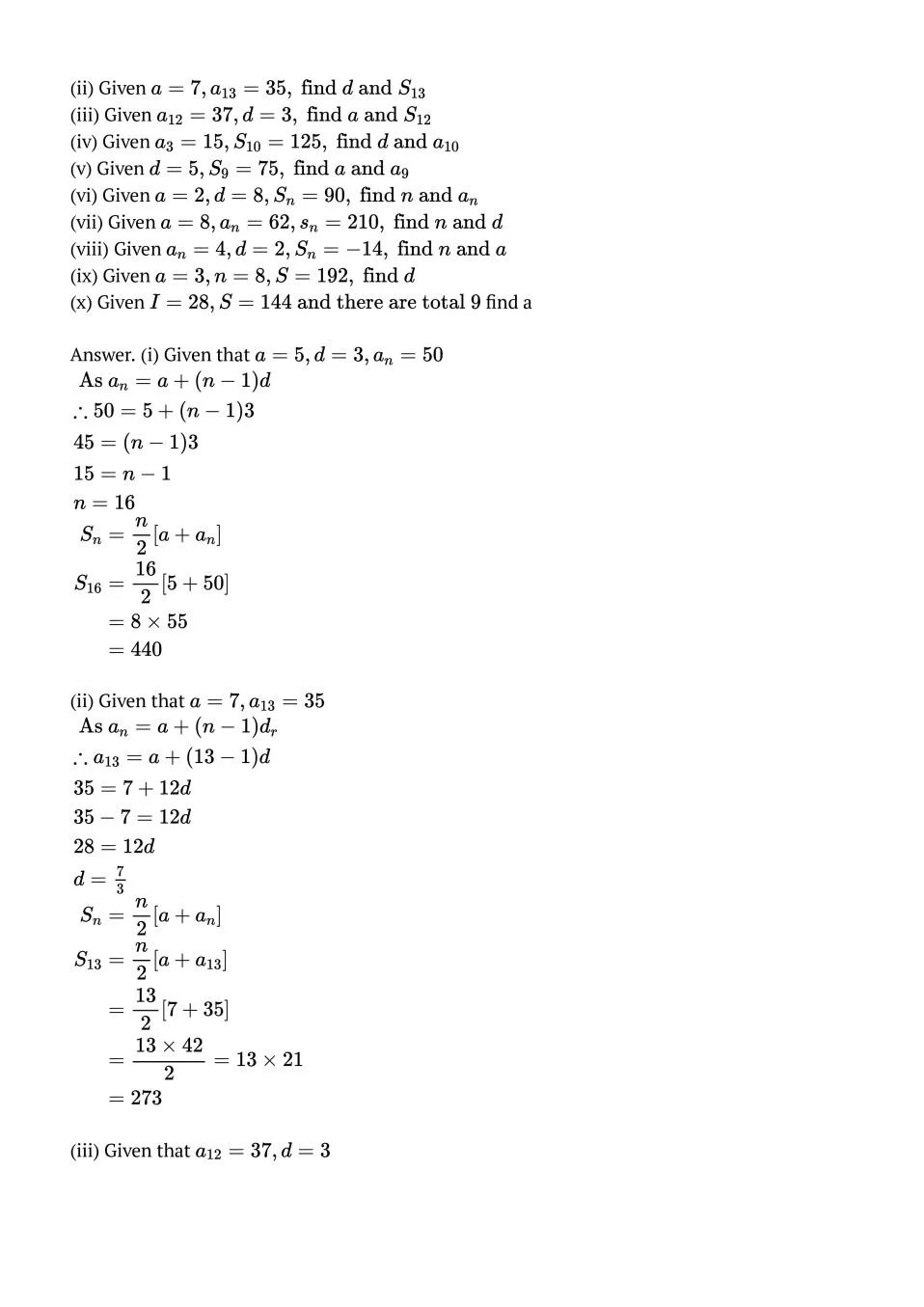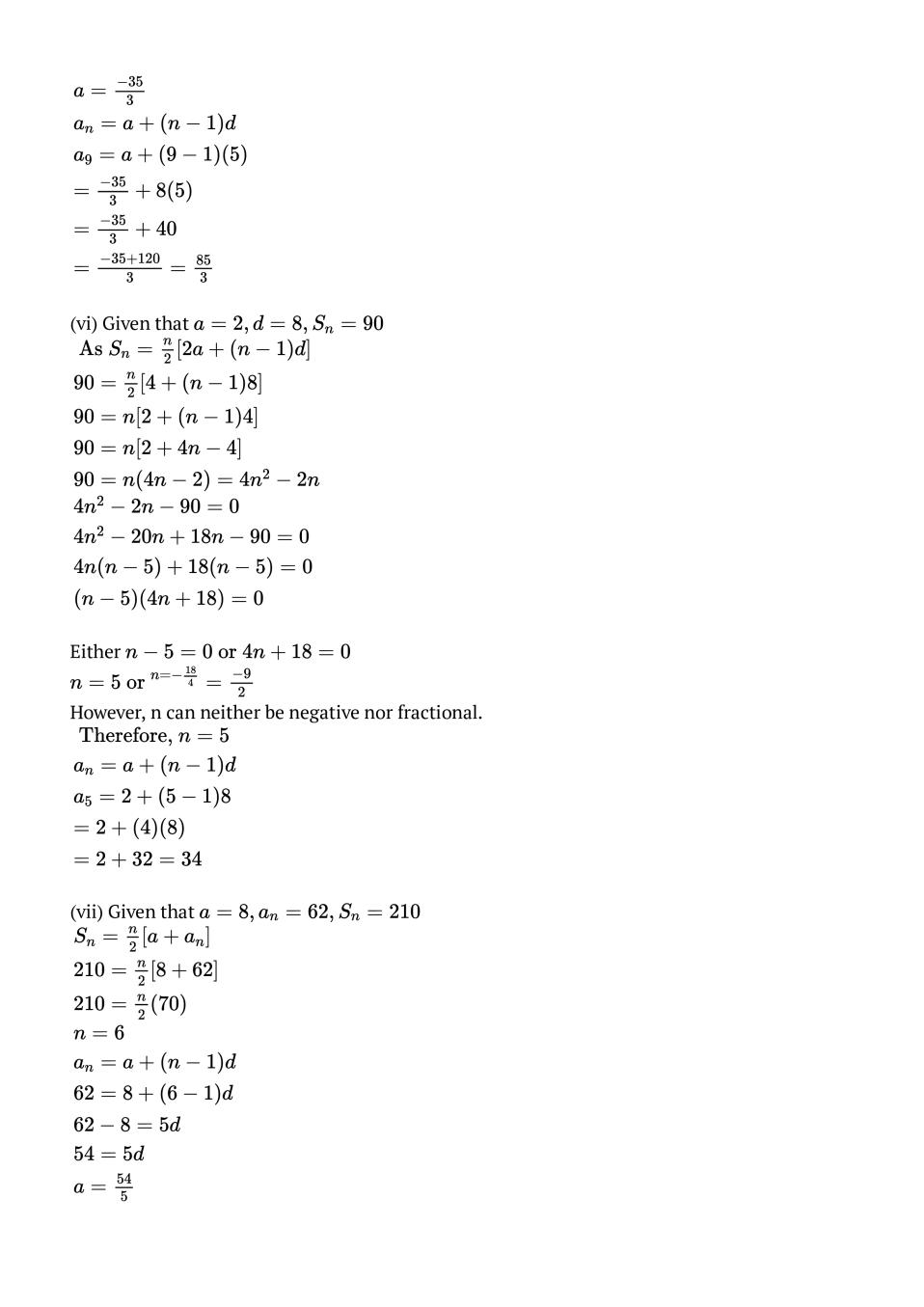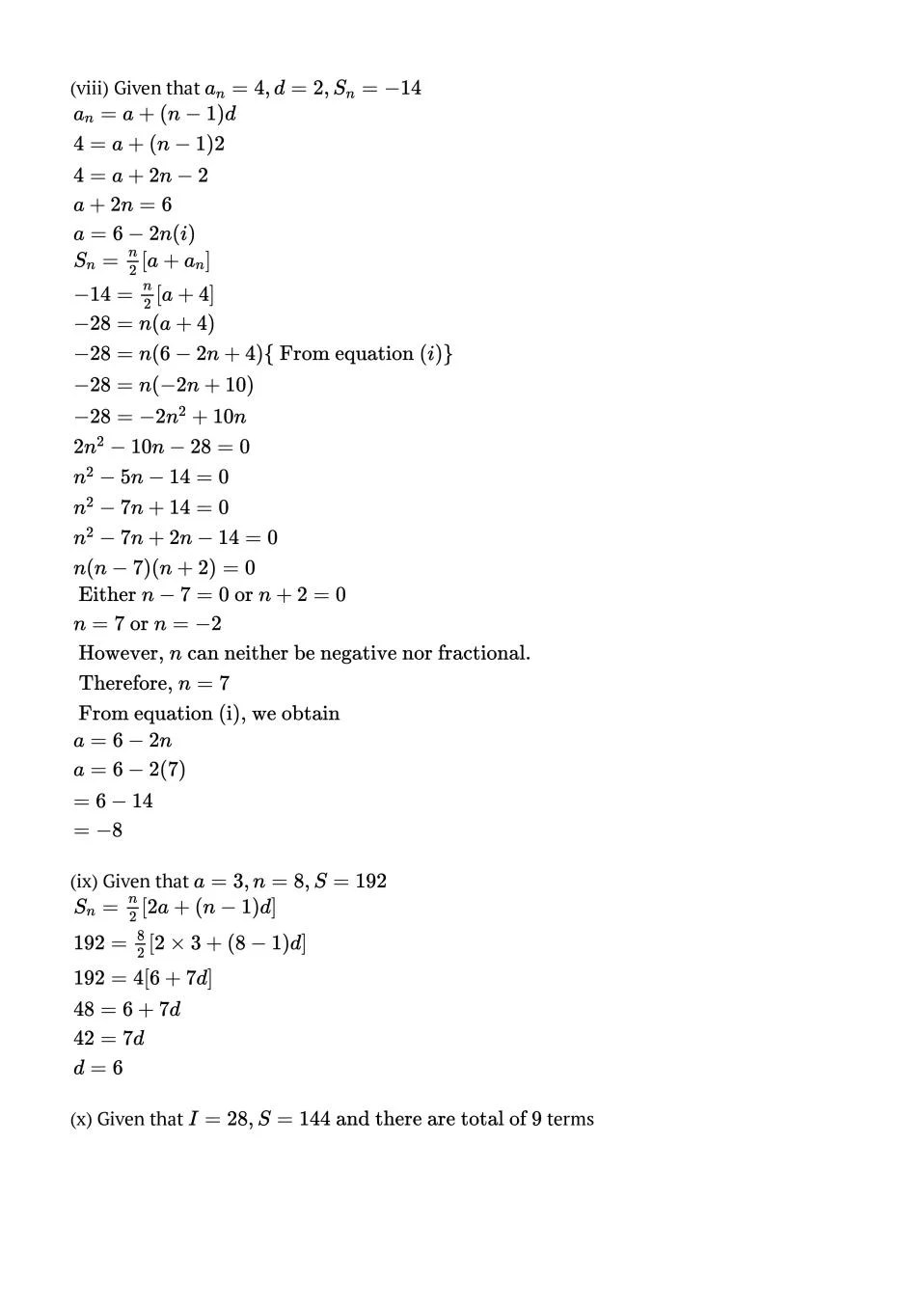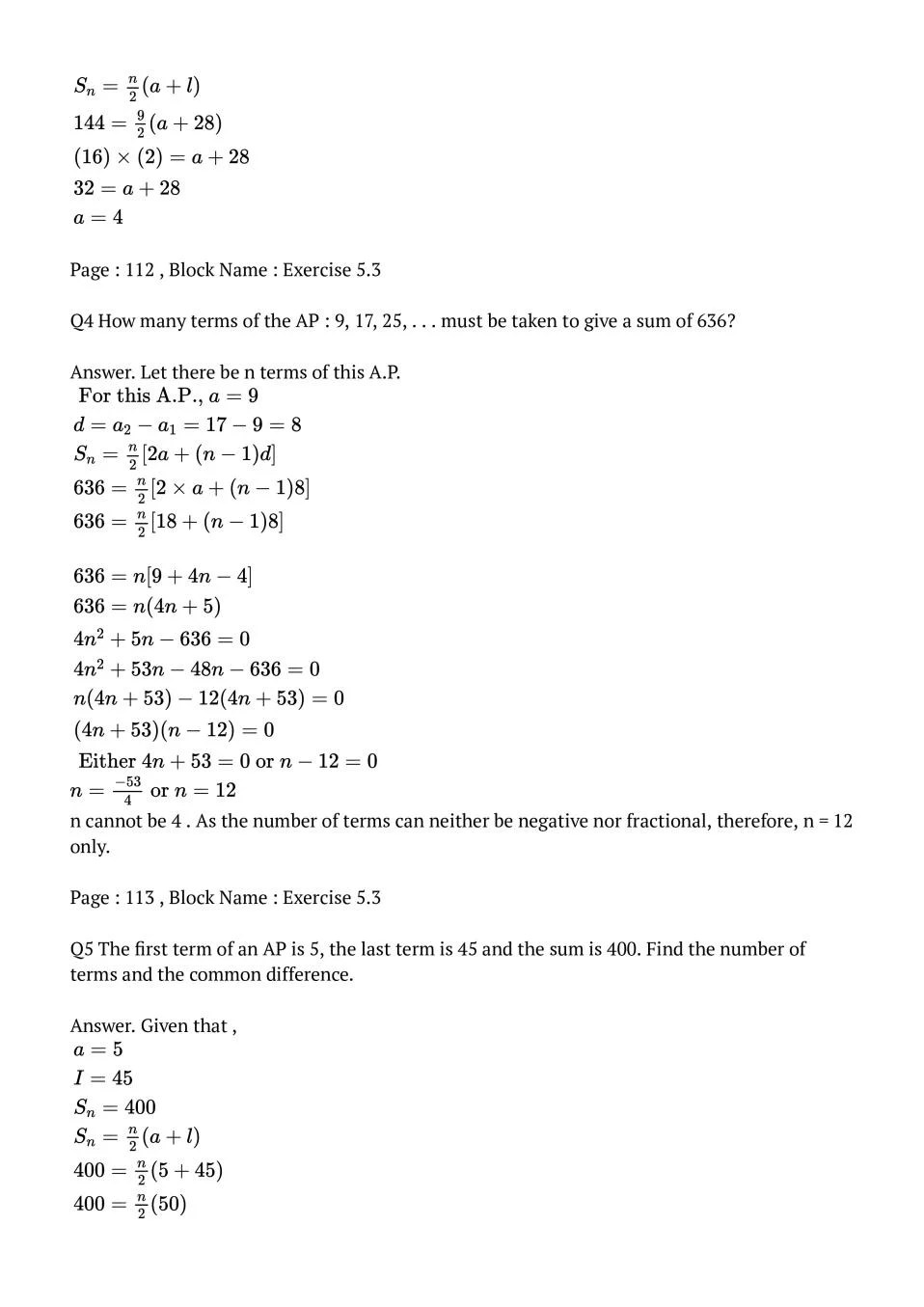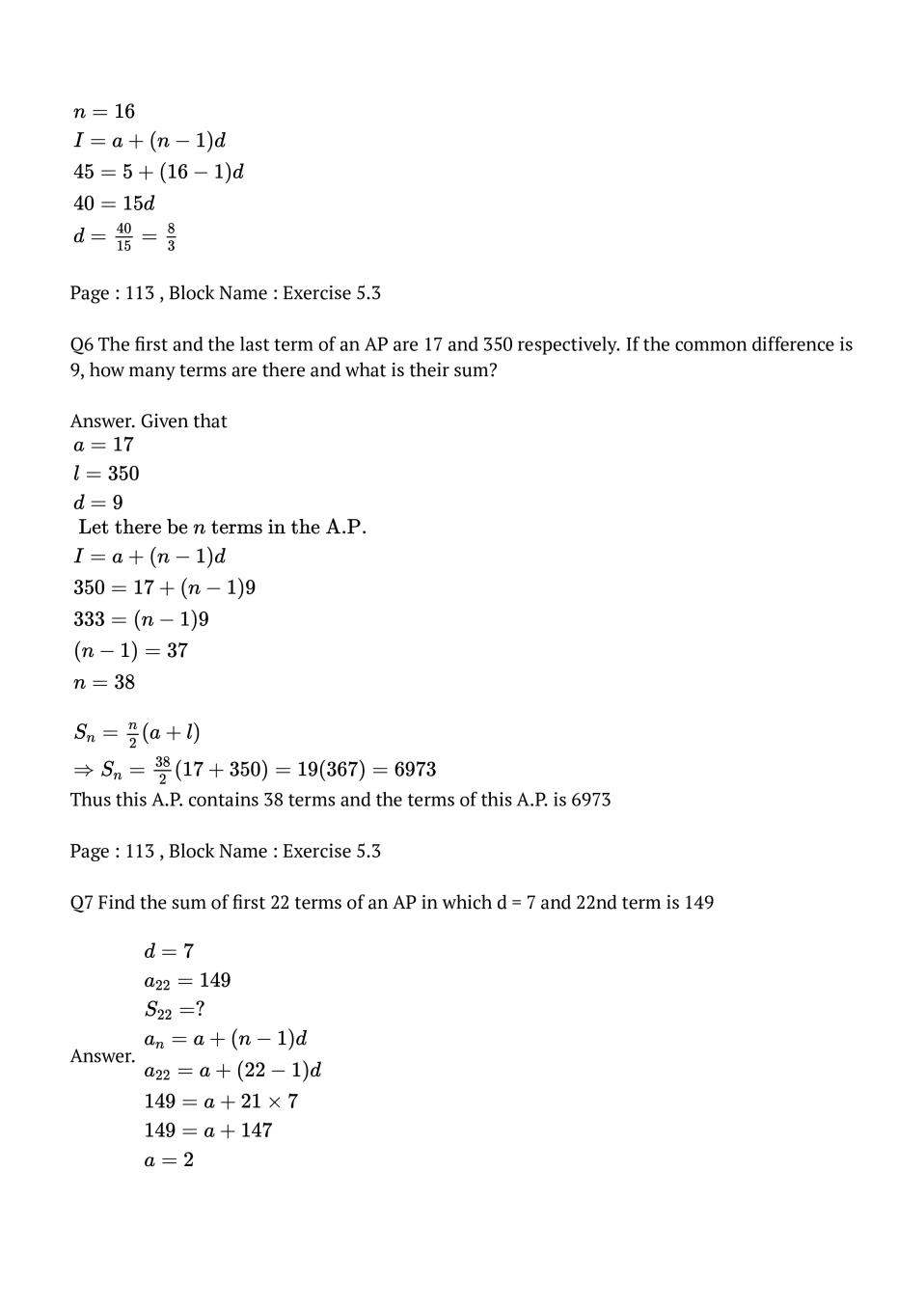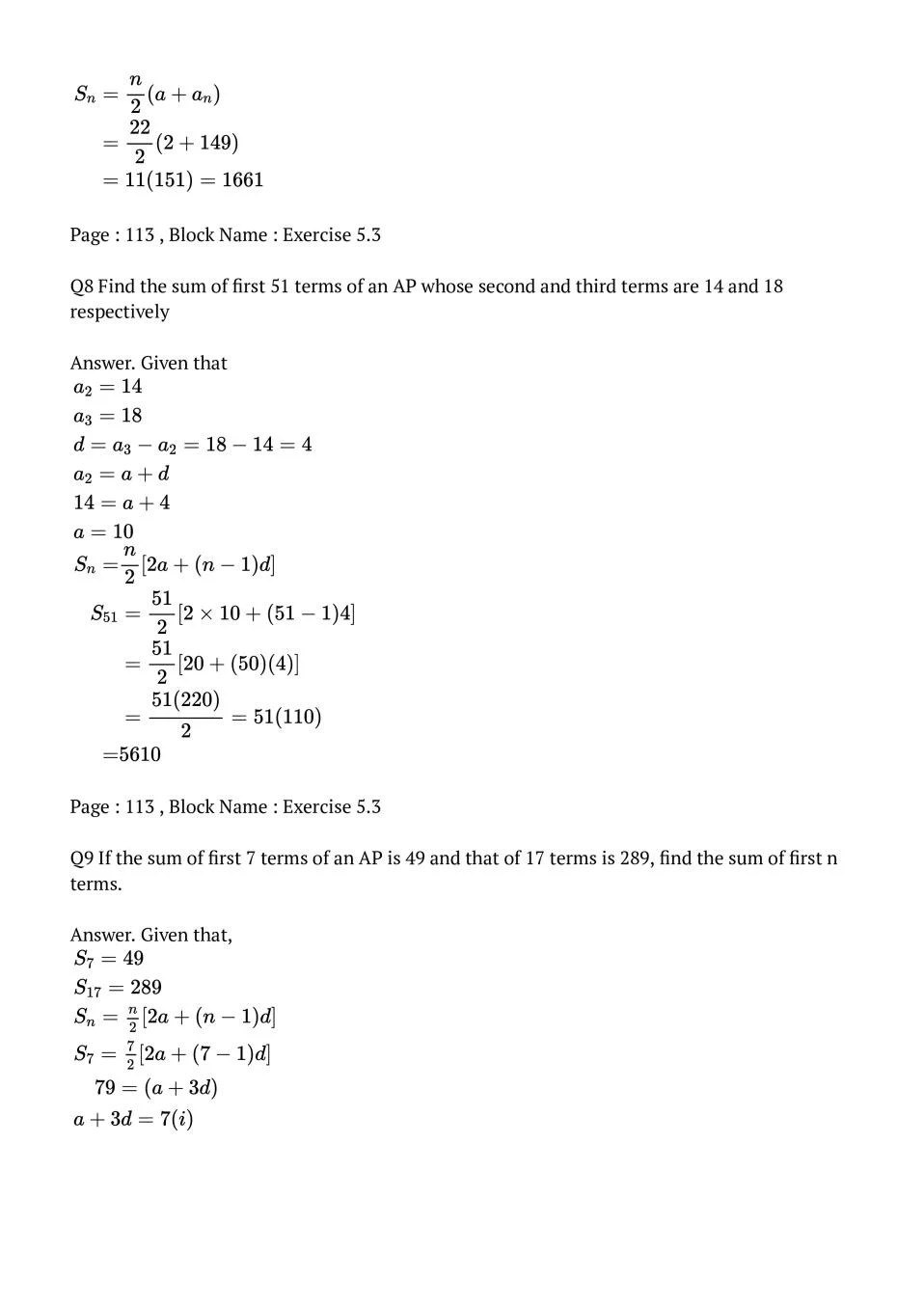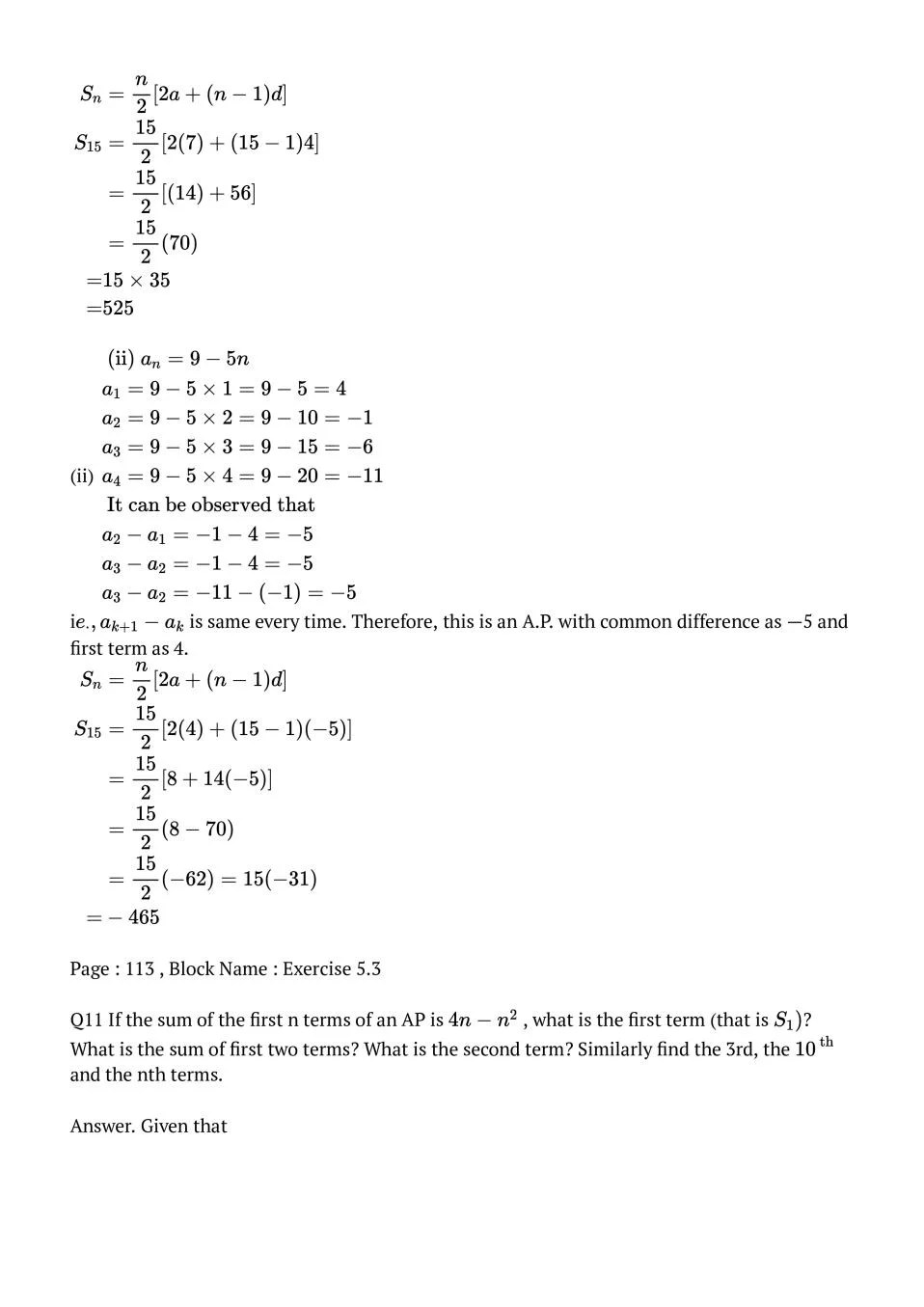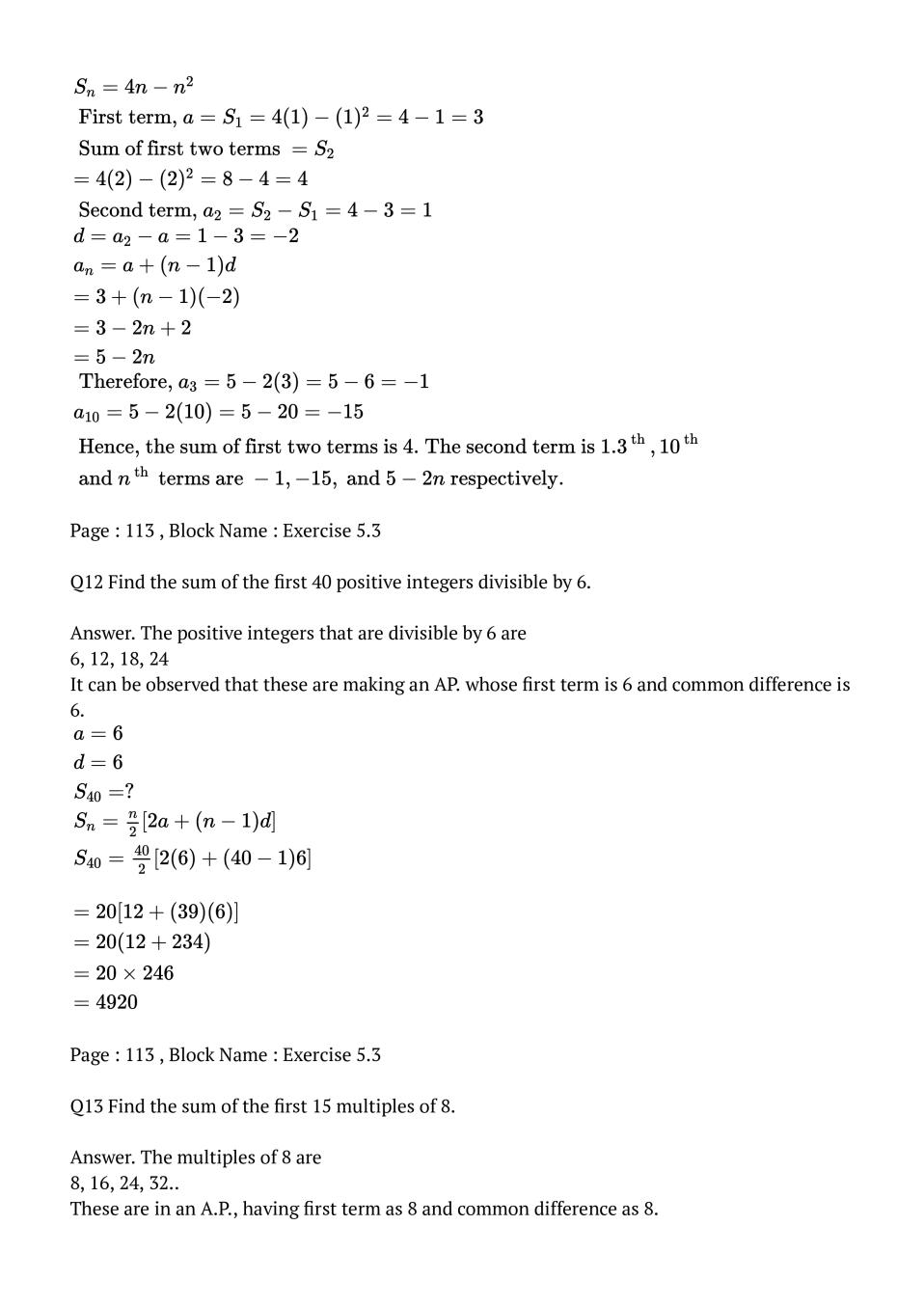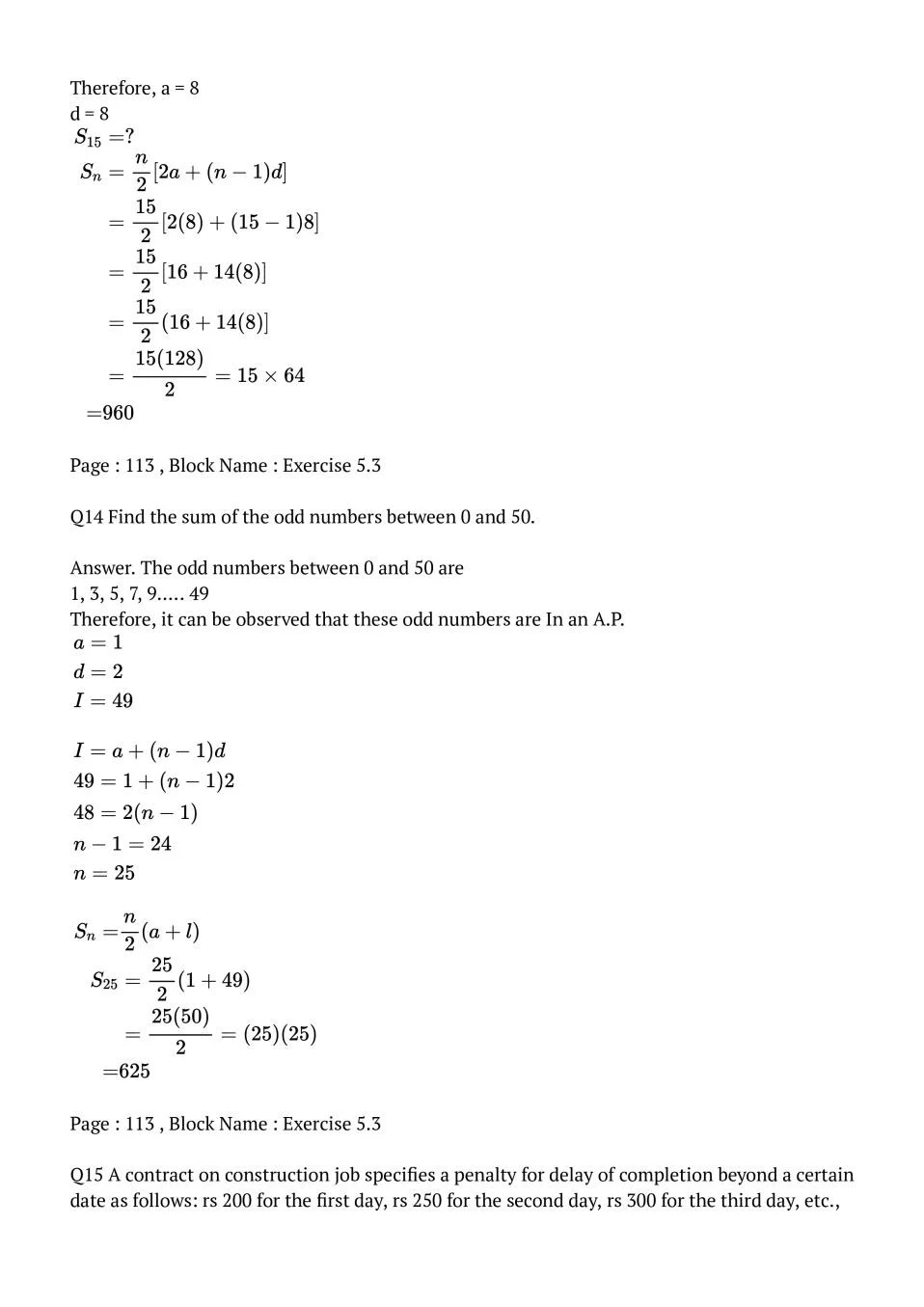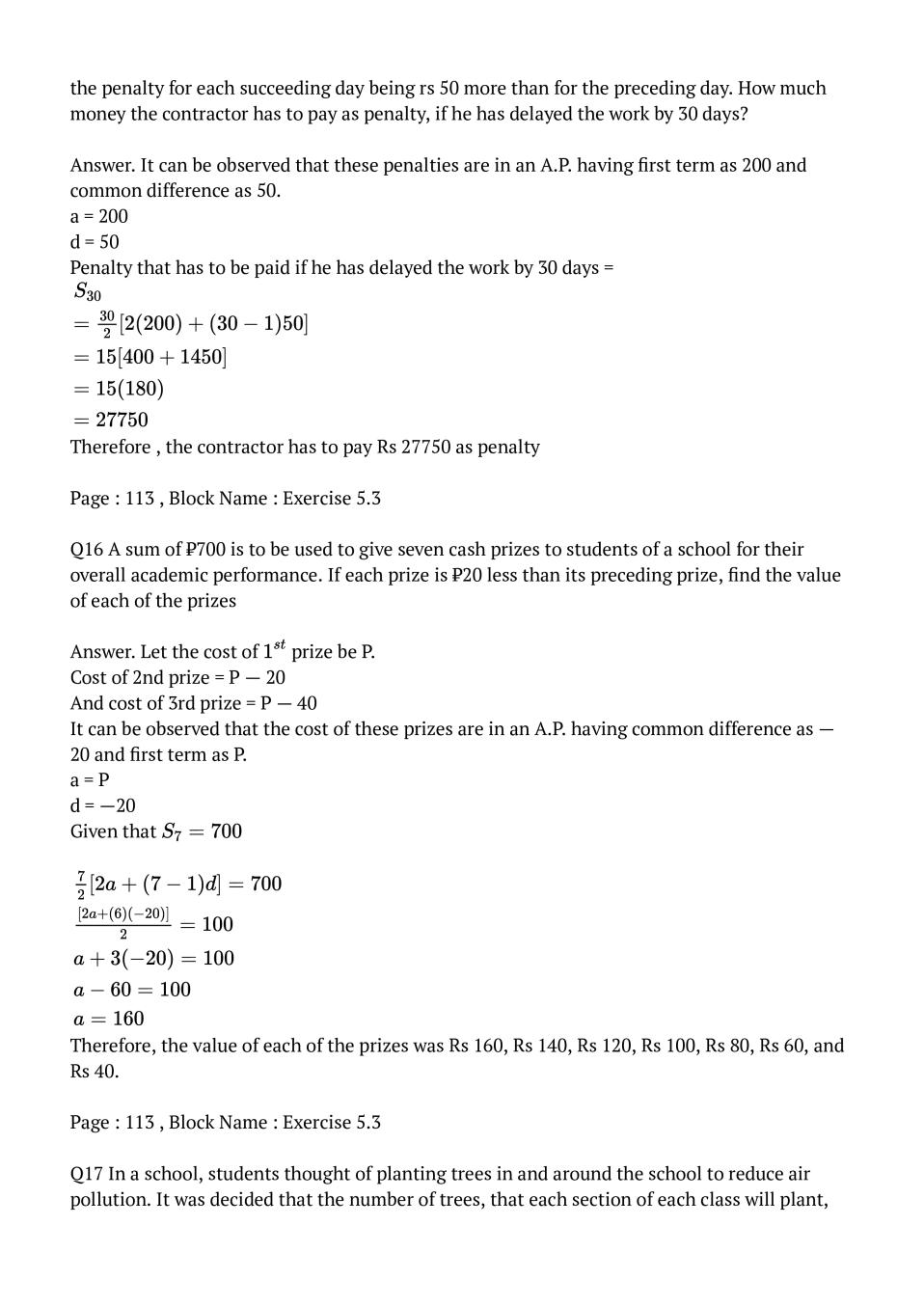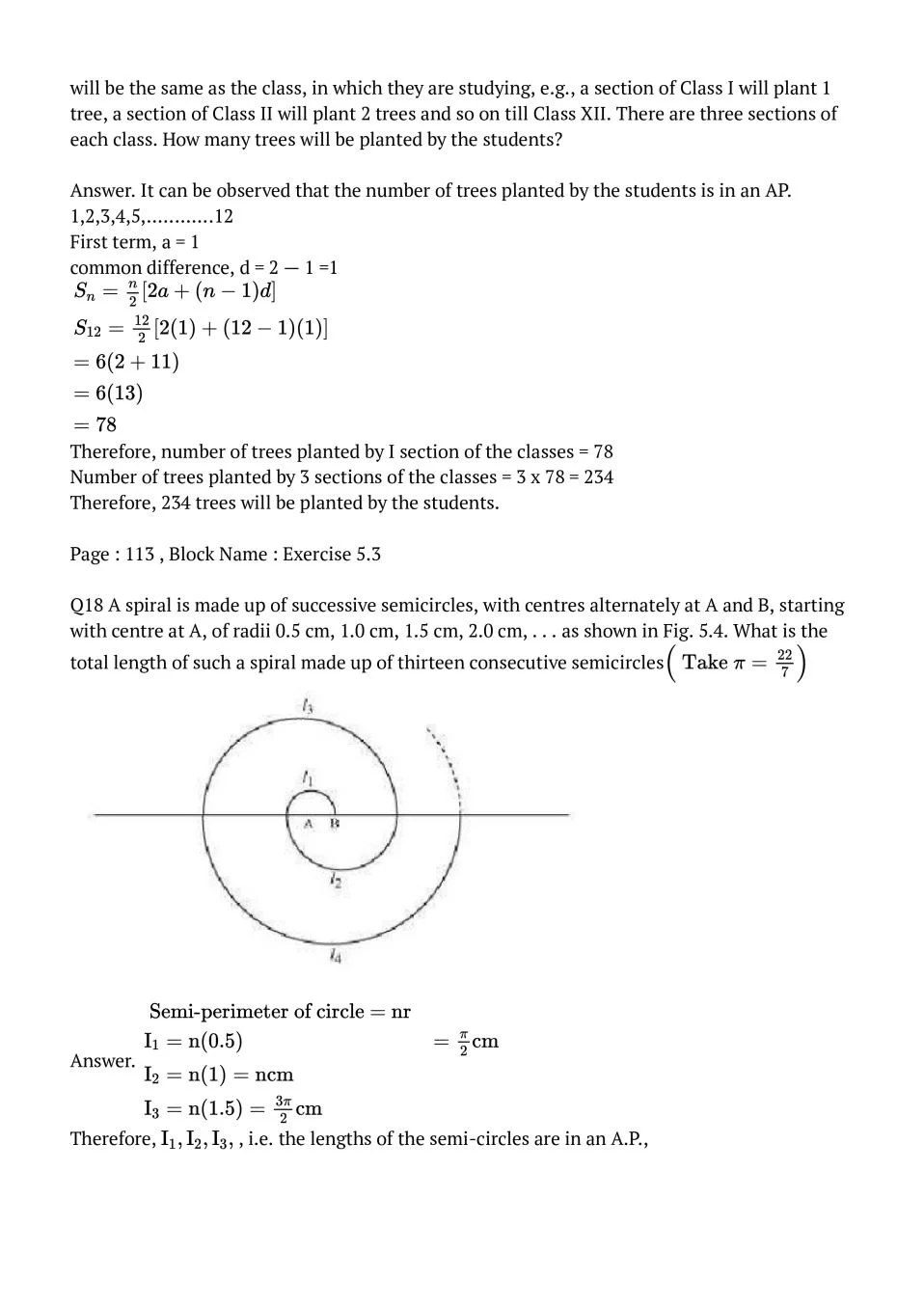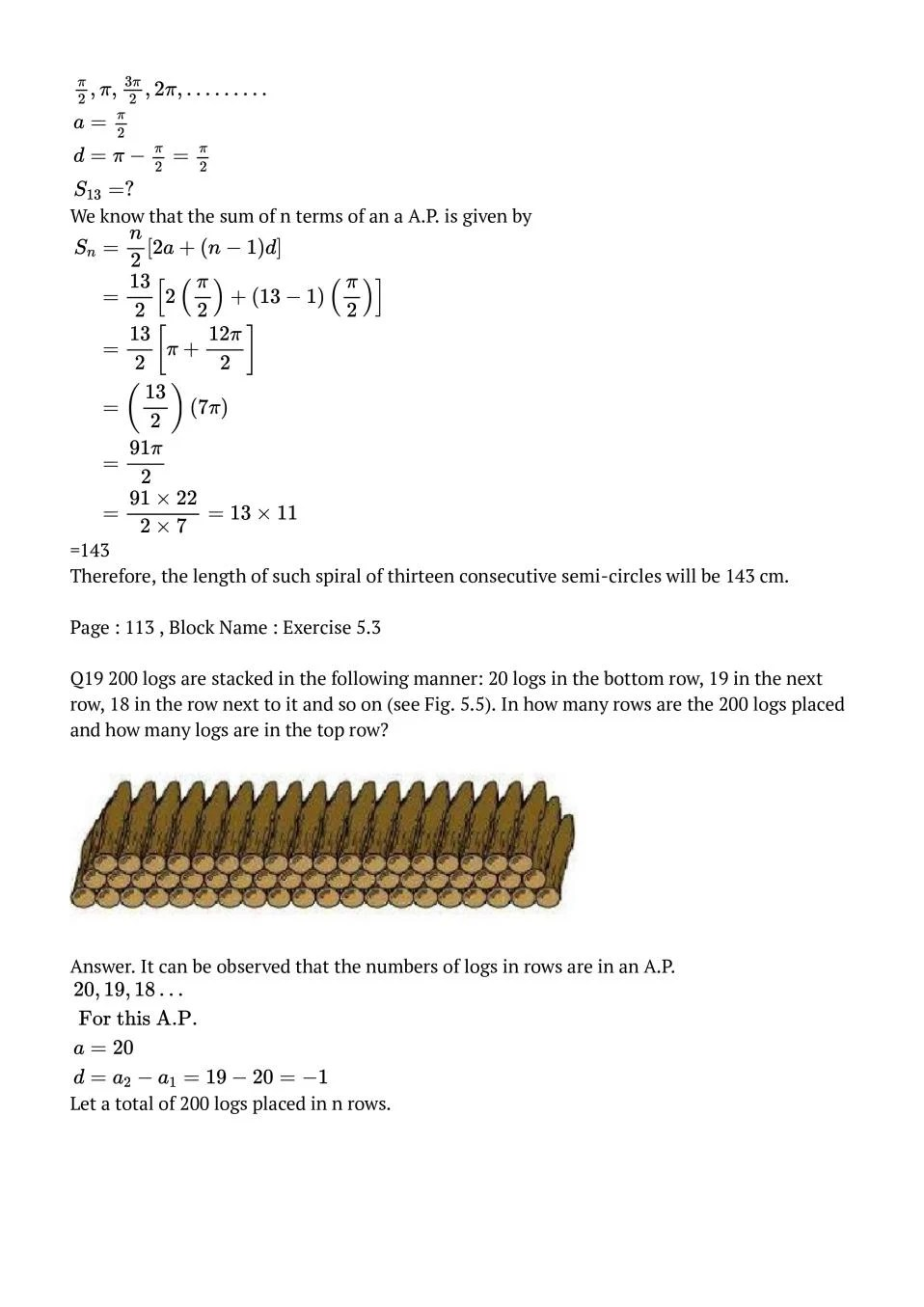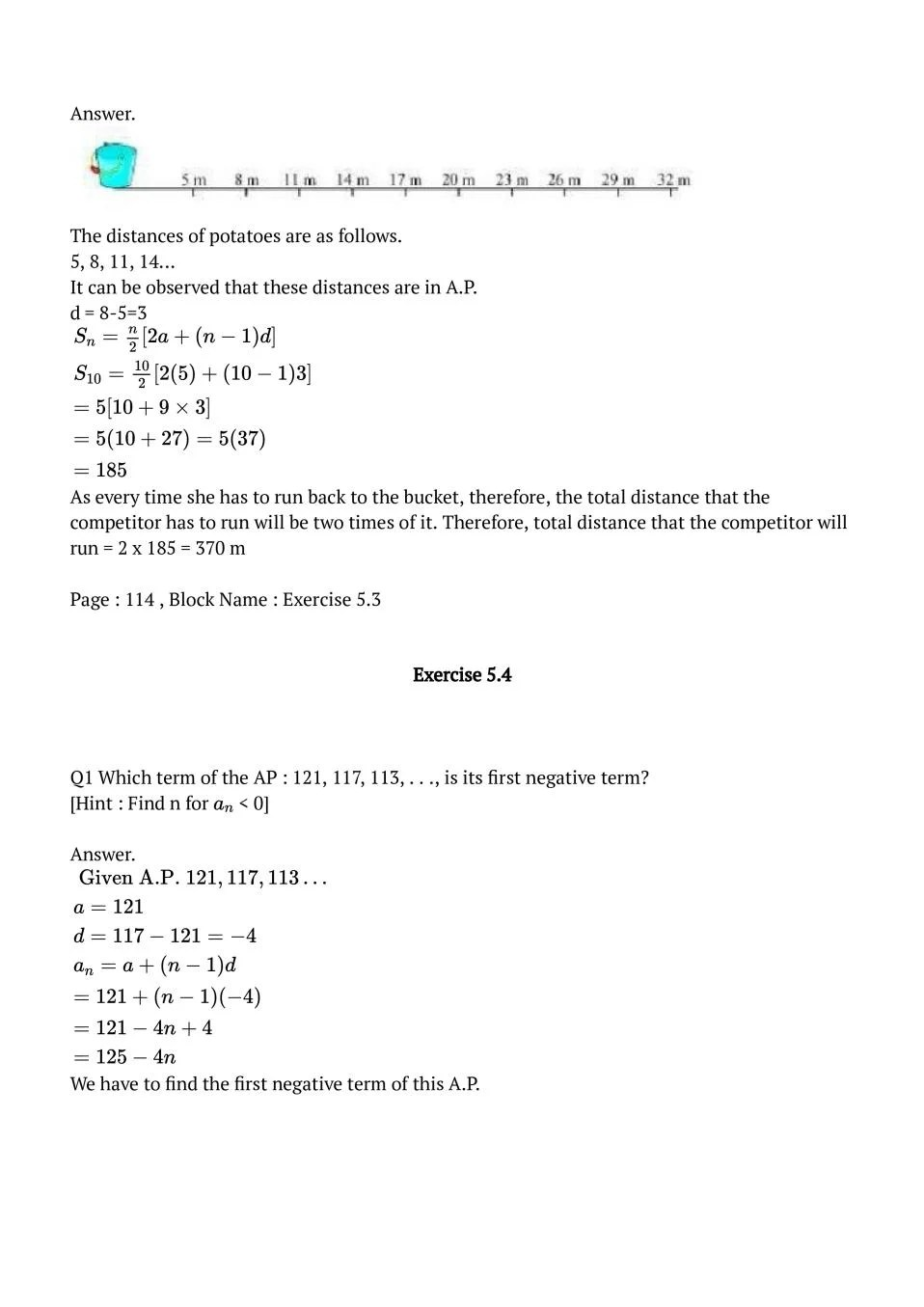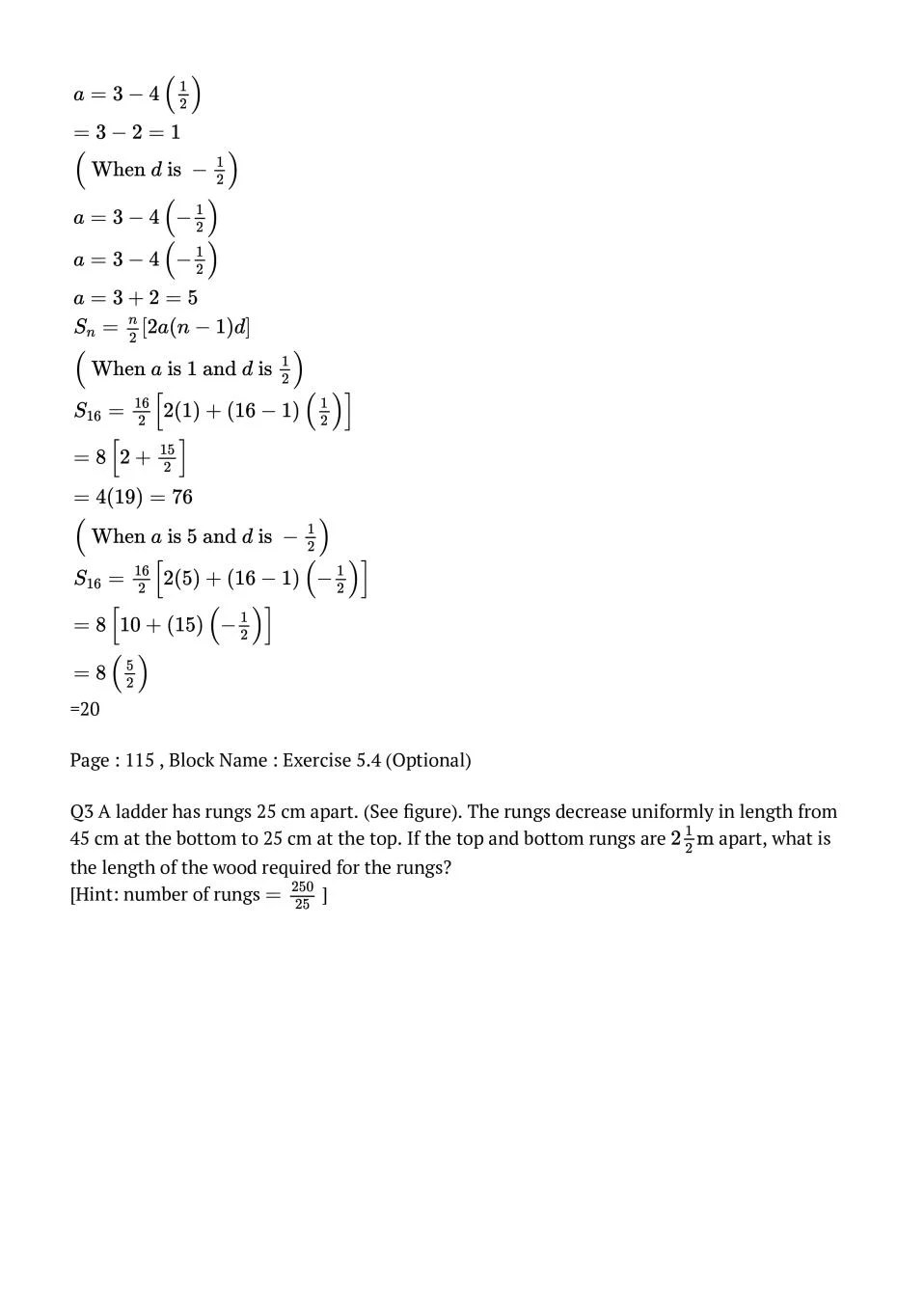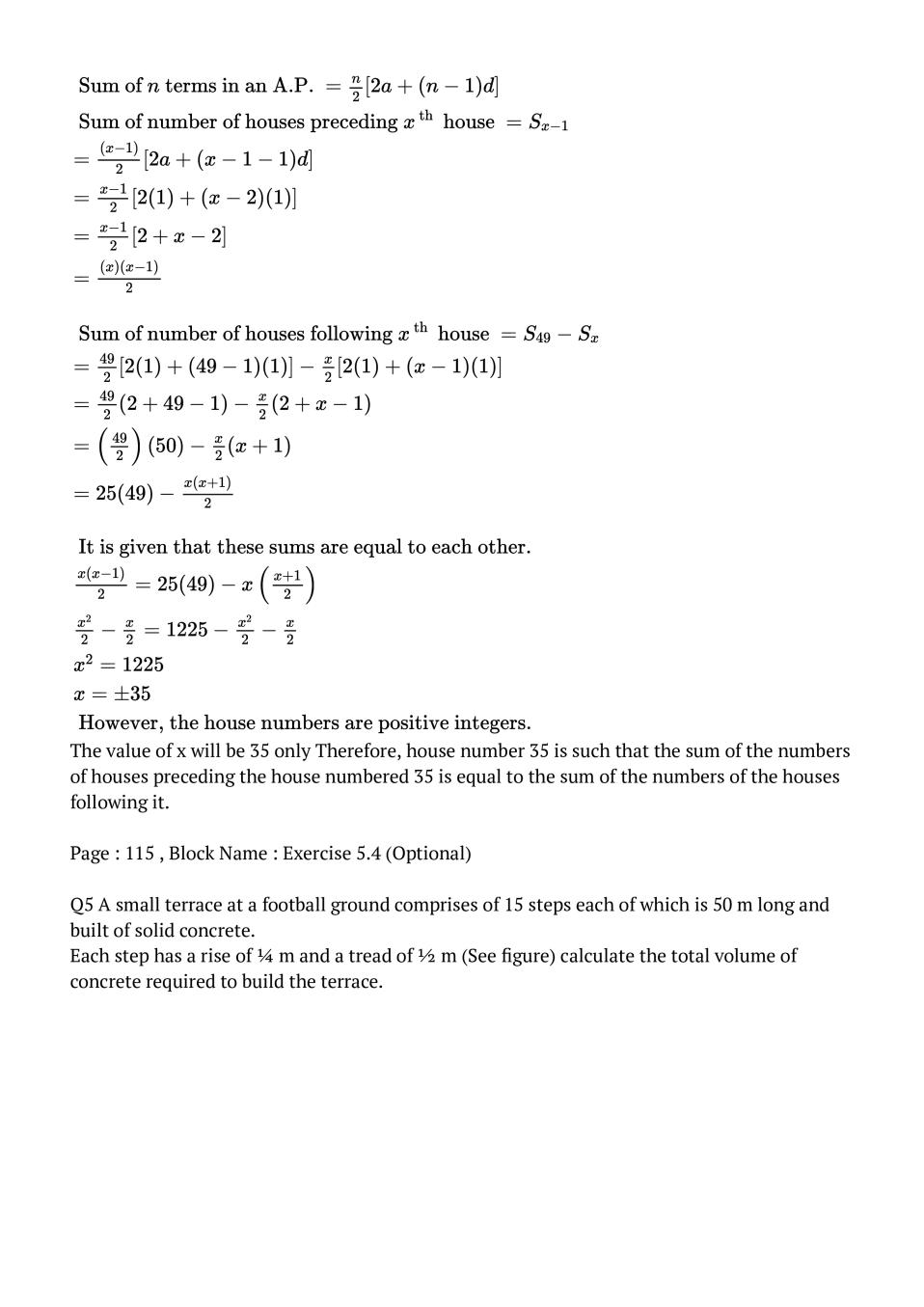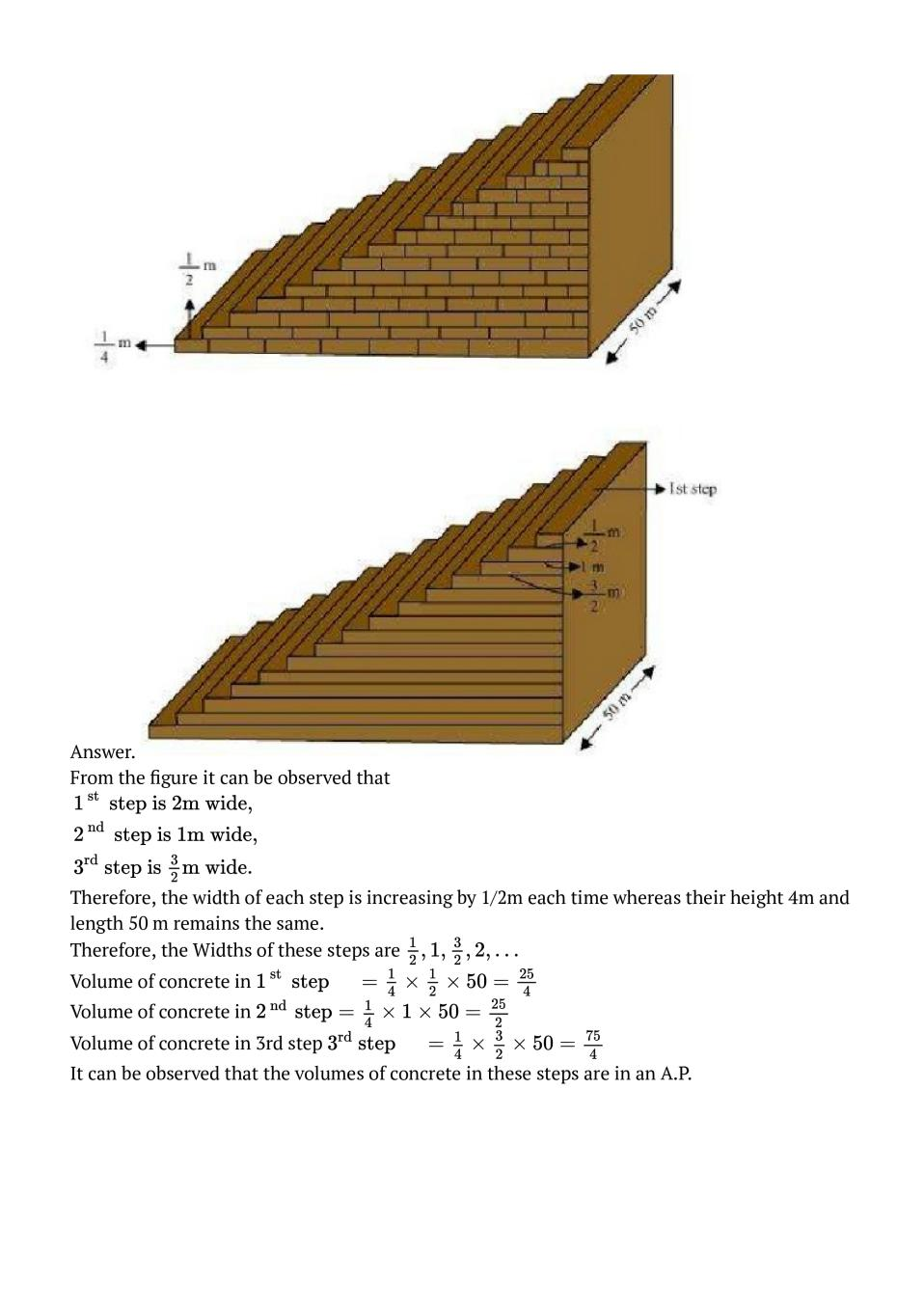### Class 10 Maths Chapter 5 NCERT Solutions

Get step by step solutions for the questions given in class 10 Maths textbook from the latest NCERT as per board exam guidelines.

### Class 10 Maths Chapter 5 Arithmetic Progressions Important Topics

5.1 Introduction
5.2 Arithmetic Progressions
5.3 nth Term of an AP
5.4 Sum of First n Terms of an AP
5.5 Summary

Exercise Covered under Maths Book Class 10 Maths Chapter 5 NCERT Solutions

Here on this page we have covered following exercises from NCERT Maths Book for Class 10 Maths Chapter 5 Arithmetic Progressions

• Exercise: 5.1
• Exercise: 5.2
• Exercise: 5.3
• Exercise: 5.4

We also suggest you to practice all the solved examples given in the NCERT book to clear your concepts on Arithmetic Progressions. You can buy NCERT Books for Class 10 Maths online for doorstep delivery.

### NCERT Solutions Class 10 Chapter Wise

If you want NCERT solutions of any topic other than Chapter 5 Arithmetic Progressions, check it from here. Maths Book by NCERT has all chapters that are in your Class 10 syllabus.

### NCERT Solutions Class 10 All Subjects

All NCERT Solutions for Subjects other than Maths are provided below:

 English Hindi Science Maths Social Science

### Other Classes NCERT Solutions

If you want NCERT solutions of any class other than Class 10, check it from here.